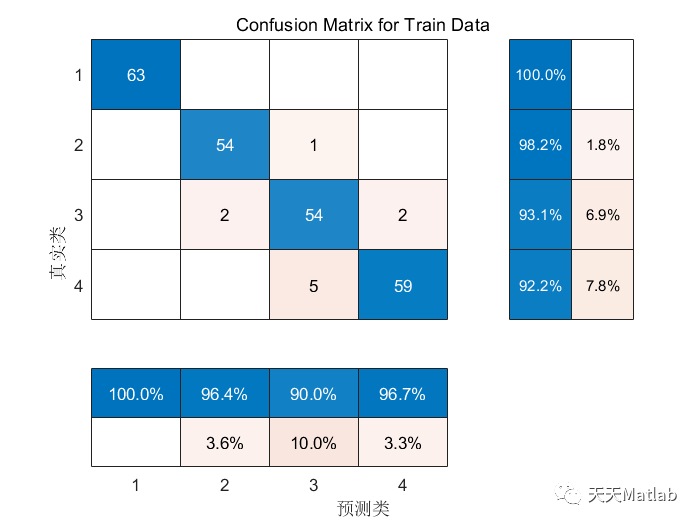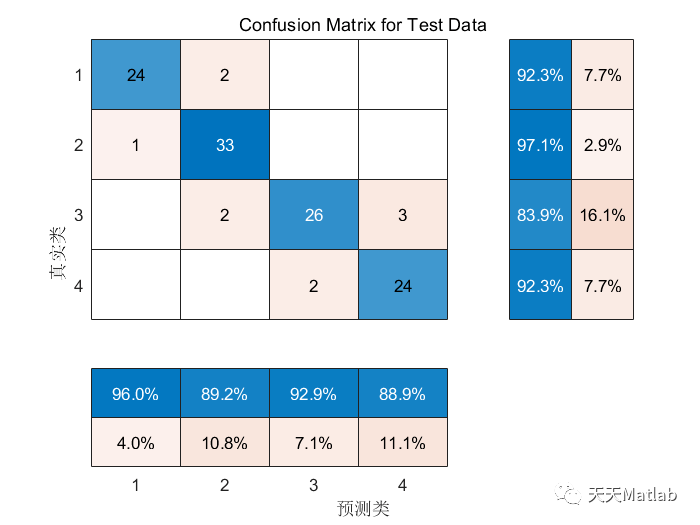# [SVM Classification] Data classification based on convolutional neural network combined with support vector machine CNN-SVM with matlab code

?About the author: A Matlab simulation developer who loves scientific research. He cultivates his mind and improves his technology simultaneously. For cooperation on MATLAB projects, please send a private message.

Personal homepage: Matlab Research Studio

Personal credo: Investigate things to gain knowledge.

For more Matlab simulation content click

Intelligent optimization algorithm Neural network prediction Radar communication Wireless sensor Power system

Signal processing Image processing Path planning Cellular automaton Drone

## Content introduction

SVM classification is a commonly used machine learning algorithm that performs well in data classification problems. However, with the rise of convolutional neural networks (CNN), researchers have begun to explore methods of combining CNN with SVM to further improve the accuracy and efficiency of data classification. In this article, we will introduce a data classification method based on convolutional neural network combined with support vector machine-CNN-SVM.

Convolutional neural network is a deep learning model widely used in the fields of image recognition and computer vision. It can effectively extract feature information in images through multi-layer convolution and pooling operations. CNN has achieved great success in image classification tasks, but its computational complexity is high and its training time is long when processing large-scale data sets. This provides us with an opportunity to introduce SVM.

Support vector machine is a binary classification model that separates data points of different categories by constructing an optimal hyperplane in the feature space. SVM has good generalization ability when processing high-dimensional data and can effectively handle large-scale data sets. However, SVM itself cannot directly process image data because image data is high-dimensional and has spatial structure. Therefore, we need to combine CNN with SVM to take full advantage of both models.

The basic idea of CNN-SVM is that during the training process, we first use CNN to extract features of the image, and then use these features as the input of SVM. Specifically, we can take the output of the last convolutional layer of CNN as a feature vector and then use SVM for classification. The advantage of this method is that we can use the feature extraction capability of CNN to convert image data into low-dimensional feature vectors, thereby reducing the dimensionality of the data. This not only reduces the computational complexity of SVM, but also improves the accuracy of classification.

In practical applications, we can use pre-trained CNN models, such as VGG16 or ResNet, to extract features of images. We then input these features into SVM for training and classification. In this way, we can make full use of CNN’s ability to train on large-scale data sets, while taking advantage of SVM’s advantages on high-dimensional data.

However, CNN-SVM also has some challenges and limitations. First, since CNN and SVM are two different models, their training processes are separated, thus requiring additional computing resources and time. Secondly, CNN-SVM may face memory and computing resource limitations when processing large-scale data sets. In addition, the performance of CNN-SVM is also affected by factors such as the selection of the CNN model and the adjustment of SVM parameters.

In summary, the data classification method based on convolutional neural network combined with support vector machine – CNN-SVM, is a potential classification algorithm. It can make full use of the advantages of CNN in image feature extraction, and at the same time, it can take advantage of the advantages of SVM in high-dimensional data. However, we need to pay attention to its computing and resource requirements when using CNN-SVM, and we need to make reasonable selection and parameter adjustment of the model. It is hoped that through further research and practice, we can further improve the performance of CNN-SVM and make it useful in a wider range of data classification problems.

## Core code

`%% Clear environment variables</code><code>warning off % Close alarm information</code><code>close all % Close open figure window</code><code>clear % Clear variables</code><code>clc % clear command line</code><code>?</code><code>%% import data</code><code>res = xlsread('dataset.xlsx');</code><code>?</code><code>%% divide the training set and test set</code><code>temp = randperm(357);</code><code>?</code><code>P_train = res(temp(1: 240), 1: 12)';</code><code>T_train = res(temp(1: 240), 13)';</code><code>M = size(P_train, 2);</code><code>?</code><code>P_test = res(temp(241: end), 1: 12)';</code><code>T_test = res(temp(241: end), 13)';</code><code>N = size(P_test, 2);</code><code>?</code><code>%% data normalization化</code><code>[p_train, ps_input] = mapminmax(P_train, 0, 1);</code><code>p_test = mapminmax('apply', P_test, ps_input );</code><code>t_train = T_train;</code><code>t_test = T_test ;</code><code>?</code><code>%% transpose to fit the model</code><code>p_train = p_train\ '; p_test = p_test';</code><code>t_train = t_train'; t_test = t_test';</code><code>?</code><code>%% Create model</code> <code>c = 10.0; % penalty factor</code><code>g = 0.01; % radial basis function parameters</code><code>cmd = ['-t 2', '-c\ ', num2str(c), '-g', num2str(g)];</code><code>model = svmtrain(t_train, p_train, cmd);`

## Operation results## ? References

 Zhang Dandan. Research on CNN classification model based on SVM and RF and its application in face detection [D]. Nanjing University of Posts and Telecommunications, 2016.

 Sun Jingyang. Design and implementation of emotion analysis and social sharing system based on ECG data [D]. Beijing University of Posts and Telecommunications, 2018.

 Wang Zheng, Li Haoyue, Xu Hongshan, et al. Emotional classification of Chinese paintings based on convolutional neural network and SVM [J]. Journal of Nanjing Normal University: Natural Science Edition, 2017, 40(3):7.DOI: 10.3969/j.issn.1001-4616.2017.03.011.

 Yang Hongyun, Huang Qiong, Sun Aizhen, et al. Rice seed image classification and recognition based on convolutional neural network and support vector machine [J]. Chinese Journal of Cereals and Oils, 2021(012):036.